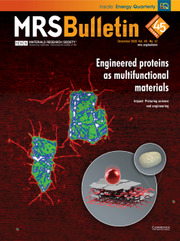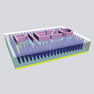Home
Hostname: page-component-99c86f546-n7x5d Total loading time: 0.289 Render date: 2021-12-07T21:46:24.739Z Has data issue: true Feature Flags: { "shouldUseShareProductTool": true, "shouldUseHypothesis": true, "isUnsiloEnabled": true, "metricsAbstractViews": false, "figures": true, "newCiteModal": false, "newCitedByModal": true, "newEcommerce": true, "newUsageEvents": true }MRS Bulletin

# Piezo-phototronic effect on optoelectronic nanodevices

Published online by Cambridge University Press:  10 December 2018

## AbstractOptoelectronic nanoscale devices have wide applications in chemical, biological, and medical technologies. Improving the performance efficiency of these devices remains a challenge. Performance is mainly dictated by the structure and characteristics of the semiconductor materials. Once a nanodevice is fabricated, its efficiency is determined. The key to improving efficiency is to control the interfaces in the device. In this article, we describe how the piezo-phototronic effect can be effectively utilized to modulate the band at the interface of a metal/semiconductor contact or a pn junction to enhance the external efficiency of many optoelectronic nanoscale devices such as photodetectors, solar cells, and light-emitting diodes (LEDs). The piezo-phototronic effect can be highly effective at enhancing the efficiency of energy conversion in today’s green and renewable energy technology without using the sophisticated nanofabrication procedures that have high cost and complexity.

## Keywords

Type
Piezotronics and Piezo-Phototronics
Information
MRS Bulletin , December 2018 , pp. 952 - 958

## Access options

Get access to the full version of this content by using one of the access options below. (Log in options will check for institutional or personal access. Content may require purchase if you do not have access.)

## References

Matterson, B.J., Lupton, J.M., Safonov, A.F., Salt, M.G., Barnes, W.L., Samuel, I.D.W., Adv. Mater. 13 (2), 123 (2001).3.0.CO;2-D>CrossRefGoogle Scholar
Kim, D.H., Cho, C.O., Roh, Y.G., Jeon, H., Park, Y.S., Cho, J., Im, J.S., Sone, C., Park, Y., Choi, W.J., Park, Q.H., Appl. Phys. Lett. 87 (20), 203508 (2005).CrossRefGoogle Scholar
Saxena, K., Jain, V.K., Mehta, D.S., Opt. Mater. 32 (1), 221 (2009).CrossRefGoogle Scholar
Reineke, S., Lindner, F., Schwartz, G., Seidler, N., Walzer, K., Lüssem, B., Leo, K., Nature 459 (7244), 234 (2009).CrossRefGoogle Scholar
Kwon, M.K., Kim, J.Y., Kim, B.H., Park, I.K., Cho, C.Y., Byeon, C.C., Park, S.J., Adv. Mater. 20 (7), 1253 (2008).CrossRefGoogle Scholar
Wang, Z.L., Wu, W., Falconi, C., MRS Bull. 43 (12), xxx (2018).CrossRefGoogle Scholar
Wang, Z.L., Piezotronics and Piezo-phototronics (Springer-Verlag, Berlin, 2012).CrossRefGoogle Scholar
Yang, Q., Guo, X., Wang, W.H., Zhang, Y., Xu, S., Lien, D.H., Wang, Z.L., ACS Nano 4 (10), 6285 (2010).CrossRefGoogle Scholar
Pan, C.F., Chen, M.X., Yu, R.M., Yang, Q., Hu, Y.F., Zhang, Y., Wang, Z.L., Adv. Mater. 28 (8), 1535 (2016).CrossRefGoogle Scholar
Qi, J.J., Lan, Y.W., Stieg, A.Z., Chen, J.H., Zhong, Y.L., Li, L.J., Chen, C.D., Zhang, Y., Wang, K.L., Nat. Commun. 6, 7430 (2015).CrossRefGoogle Scholar
Chen, M.X., Pan, C.F., Zhang, T.P., Li, X.Y., Liang, R.R., Wang, Z.L., ACS Nano 10 (6), 6074 (2016).CrossRefGoogle Scholar
Hu, Y.F., Chang, Y.L., Fei, P., Snyder, R.L., Wang, Z.L., ACS Nano 4 (2), 1234 (2010).CrossRefGoogle Scholar
Wu, W.Z., Wen, X.N., Wang, Z.L., Science 340, 952 (2013).CrossRefGoogle Scholar
Wang, Z.L., Wu, W.Z., Natl. Sci. Rev. 1, 62 (2014).CrossRefGoogle Scholar
Wu, W.Z., Wang, L., Li, Y.L., Zhang, F., Lin, L., Niu, S.M., Chenet, D., Zhang, X., Hao, Y.F., Heinz, T.F., Hone, J., Wang, Z.L., Nature 514, 470 (2014).CrossRefGoogle Scholar
Yu, R.M., Niu, S.M., Pan, C.F., Wang, Z.L., Nano Energy 14, 312 (2015).CrossRefGoogle Scholar
Yu, R.M., Wang, X.F., Wu, W.Z., Pan, C.F., Bando, Y., Fukata, N., Hu, Y.F., Peng, W.B., Ding, Y., Wang, Z.L., Adv. Funct. Mater. 25, 5277 (2015).CrossRefGoogle Scholar
Wang, Z.L., J. Phys. Chem. Lett. 1, 1388 (2010).CrossRefGoogle Scholar
Zhao, Z.F., Pu, X., Han, C.B., Du, C.H., Li, L.X., Jiang, C.Y., Hu, W.G., Wang, Z.L., ACS Nano 9 (8), 8578 (2015).CrossRefGoogle Scholar
Pradel, K.C., Wu, W.Z., Zhou, Y.S., Wen, X.N., Ding, Y., Wang, Z.L., Nano Lett. 13 (6), 2647 (2013).CrossRefGoogle Scholar
Yu, R.M., Wang, X.F., Peng, W.B., Wu, W.Z., Ding, Y., Li, S.T., Wang, Z.L., ACS Nano 9, 9822 (2015).CrossRefGoogle Scholar
Yu, R.M., Dong, L.N., Pan, C.F., Niu, S.M., Liu, H.F., Liu, W., Chua, S.J., Chi, D.Z., Wang, Z.L., Adv. Mater. 24, 3532 (2012).CrossRefGoogle Scholar
Dong, L., Niu, S., Pan, C.F., Yu, R.M., Zhang, Y., Wang, Z.L., Adv. Mater. 24, 5470 (2012).CrossRefGoogle Scholar
Zhang, F., Ding, Y., Zhang, Y., Zhang, X.L., Wang, Z.L., ACS Nano 6 (10), 9229 (2012).CrossRefGoogle Scholar
Han, X., Du, W.M., Yu, R.M., Pan, C.F., Wang, Z.L., Adv. Mater. 27, 7963 (2015).CrossRefGoogle Scholar
Yu, R.M., Pan, C.F., Hu, Y.F., Li, L., Liu, H.F., Liu, W., Chua, S.J., Chi, D.Z., Wang, Z.L., Nano Res. 6, 758 (2013).CrossRefGoogle Scholar
Dai, Y.J., Wang, X.F., Peng, W.B., Wu, C.S., Ding, Y., Dong, K., Wang, Z.L., Nano Energy 44, 311 (2018).CrossRefGoogle Scholar
Dai, Y.J., Wang, X.F., Peng, W.B., Zou, H.Y., Yu, R.M., Ding, Y., Wu, C.S., Wang, Z.L., ACS Nano 11, 7118 (2017).CrossRefGoogle Scholar
Chen, M.X., Zhao, B., Hu, G.F., Fang, X.S., Wang, H., Wang, L., Luo, J., Han, X., Wang, X.D., Pan, C.F., Wang, Z.L., Adv. Funct. Mater. 27, 1700264 (2018).Google Scholar
Dai, G.Z., Zou, H.Y., Wang, X.F., Zou, Y.K., Wang, P.H., Ding, Y., Zhang, Y., Yang, J.L., Wang, Z.L., ACS Photonics 4 (10), 2495 (2017).CrossRefGoogle Scholar
Zhang, K., Peng, M.Z., Wu, W., Guo, J.M., Gao, G.Y., Liu, Y.D., Kou, J.Z., Wen, R.M., Lei, Y., Yu, A.F., Zhang, Y., Zhai, J.Y., Wang, Z.L., Mater. Horiz. 4, 274 (2017).CrossRefGoogle Scholar
Zhu, L.P., Wang, L.F., Xue, F., Chen, L.B., Fu, J.Q., Feng, X.L., Li, T.F., Wang, Z.L., Adv. Sci. 4, 1600185 (2017).CrossRefGoogle Scholar
Tang, H., Liu, B., Wang, T., J. Phys. D Appl. Phys. 48, 025101 (2015).CrossRefGoogle Scholar
Pan, C.F., Niu, S.M., Ding, Y., Dong, L., Yu, R.M., Liu, Y., Zhu, G., Wang, Z.L., Nano Lett. 12, 3302 (2012).CrossRefGoogle Scholar
Jiang, C.Y., Jing, L., Huang, X., Liu, M.M., Du, C.H., Liu, T., Pu, X., Hu, W.G., Wang, Z.L., ACS Nano 11, 9405 (2017).CrossRefGoogle Scholar
Zou, H.Y., Li, X.G., Peng, W.B., Wu, W.Z., Yu, R.M., Wu, C.S., Ding, W.B., Hu, F., Liu, R.Y., Zi, Y.L., Wang, Z.L., Adv. Mater. 29, 1701412 (2017).CrossRefGoogle Scholar
Hu, G.F., Guo, W.X., Yang, X.N., Zhou, R.R., Pan, C.F., Wang, Z.L., Nano Energy 23, 27 (2016).CrossRefGoogle Scholar
Wen, X.N., Wu, W.Z., Wang, Z.L., Nano Energy 2, 1093 (2013).CrossRefGoogle Scholar
Zhu, L.P., Wang, L.F., Pan, C.F., Chen, L.B., Xue, F., Chen, B.D., Yang, L.J., Su, L., Wang, Z.L., ACS Nano 11 (2), 1894 (2017).CrossRefGoogle Scholar
Wang, Z.L., Nano Today 5 (6), 540 (2010).CrossRefGoogle Scholar
Yang, Q., Wang, W.H., Xu, S., Wang, Z.L., Nano Lett. 11 (9), 4012 (2011).CrossRefGoogle Scholar
Liu, Y., Niu, S.M., Yang, Q., Klein, B.D.B., Zhou, Y.S., Wang, Z.L., Adv. Mater. 26 (42), 7209 (2015).CrossRefGoogle Scholar
Yang, Q., Liu, Y., Pan, C.F., Chen, J., Wen, X.N., Wang, Z.L., Nano Lett. 13 (2), 607 (2013).CrossRefGoogle Scholar
Wang, C.F., Bao, R.R., Zhao, K., Zhang, T.P., Dong, L., Pan, C.F., Nano Energy 14, 364 (2015).CrossRefGoogle Scholar
Que, M.L., Wang, X.D., Peng, Y.Y., Pan, C.F., Chin. Phys. B 26 (6), 067301 (2017).CrossRefGoogle Scholar
Zhang, T.P., Liang, R.R., Dong, L., Wang, J., Xu, J., Pan, C.F., Nano Res. 8 (8), 2676 (2015).CrossRefGoogle Scholar
Pan, C.F., Zhu, J., J. Mater. Chem. 19, 869 (2009).CrossRefGoogle Scholar
Li, X.Y., Liang, R.R., Tao, J., Xu, Q.M., Peng, Z.C., Han, X., Wang, X.D., Wang, C.F., Zhu, J., Pan, C.F., Wang, Z.L., ACS Nano 11 (4), 3883 (2017).CrossRefGoogle Scholar
Bao, R.R., Wang, C.F., Peng, Z.C., Ma, C., Dong, L., Pan, C.F., ACS Photonics 4, 1344 (2017).CrossRefGoogle Scholar
Wang, X.F., Peng, W.B., Yu, R.M., Zou, H.Y., Dai, Y.J., Zi, Y.L., Wu, C.S., Li, S.T., Wang, Z.L., Nano Lett. 17, 3718 (2017).CrossRefGoogle Scholar
Huang, X., Jiang, C.Y., Du, C.H., Jing, L., Liu, M.M., Hu, W.G., Wang, Z.L., ACS Nano 10, 11420 (2016).CrossRefGoogle Scholar
Hua, Q.L., Sun, J.L., Liu, H.T., Bao, R.R., Yu, R.M., Zhai, J.Y., Pan, C.F., Wang, Z.L., Nat. Commun. 9, 244 (2018).CrossRefGoogle Scholar
Zhao, X.L., Hua, Q.L., Yu, R.M., Zhang, Y., Pan, C.F., Adv. Electron. Mater. 1, 1500142 (2015).CrossRefGoogle Scholar
Li, X.Y., Chen, M.X., Yu, R.M., Zhang, T.P., Song, D.S., Liang, R.R., Zhang, Q.L., Cheng, S.B., Dong, L., Pan, A.L., Wang, Z.L., Zhu, J., Pan, C.F., Adv. Mater. 27, 4447 (2015).CrossRefGoogle Scholar
Wang, C.F., Peng, D.F., Zhao, J., Bao, R.R., Li, T.F., Tian, L., Dong, L., Shen, C.Y., Pan, C.F., Small 12 (41), 5734 (2016).CrossRefGoogle ScholarPubMed
Bao, R.R., Wang, C.F., Dong, L., Shen, C.Y., Zhao, K., Pan, C.F., Nanoscale 8, 8078 (2016).CrossRefGoogle Scholar
Pan, C.F., Dong, L., Zhu, G., Niu, S.M., Yu, R.M., Yang, Q., Liu, Y., Wang, Z.L., Nat. Photonics 7, 752 (2013).CrossRefGoogle Scholar
Bao, R.R., Wang, C.F., Dong, L., Yu, R.M., Zhao, K., Wang, Z.L., Pan, C.F., Adv. Funct. Mater. 25, 2884 (2015).CrossRefGoogle Scholar

# Send article to Kindle

Note you can select to send to either the @free.kindle.com or @kindle.com variations. ‘@free.kindle.com’ emails are free but can only be sent to your device when it is connected to wi-fi. ‘@kindle.com’ emails can be delivered even when you are not connected to wi-fi, but note that service fees apply.

Find out more about the Kindle Personal Document Service.

Piezo-phototronic effect on optoelectronic nanodevices
Available formats
×

# Send article to Dropbox

To send this article to your Dropbox account, please select one or more formats and confirm that you agree to abide by our usage policies. If this is the first time you use this feature, you will be asked to authorise Cambridge Core to connect with your <service> account. Find out more about sending content to Dropbox.

Piezo-phototronic effect on optoelectronic nanodevices
Available formats
×

# Send article to Google Drive

To send this article to your Google Drive account, please select one or more formats and confirm that you agree to abide by our usage policies. If this is the first time you use this feature, you will be asked to authorise Cambridge Core to connect with your <service> account. Find out more about sending content to Google Drive.

Piezo-phototronic effect on optoelectronic nanodevices
Available formats
×
×